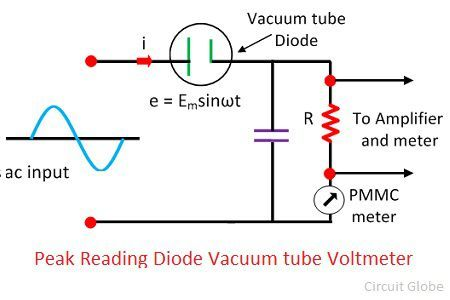# Vacuum Tube Voltmeter (VTVM)

Definition: The voltmeter which uses the vacuum tube for amplifying the measurand AC and DC voltage is known as the vacuum tube voltmeter (VTM). The vacuum tube increases the sensitivity of the voltmeter because of which it can detect the signal of very weak strength.

The electronic voltmeter is used for measuring the voltage of direct, RMS and peak voltage of the system. The vacuum tube has many advantages like they have high input impedance, wide frequency range and high sensitivity.

The most significant advantage of the VTM is that it can draw very less current as compared to the other meter. In VTM, the measuring signal is directly supplied to the vacuum tube of the meter. The vacuum tube is amplifying the signal and pass it to the deflecting meter.

## Types of Vacuum tube Voltmeter

The Vacuum Tube Voltmeter is classified into the following types.

1. Diode Type
• Peak Reading Diode Vacuum Tube Voltmeter
2. Single Triode
3. Balanced Triode Type
4. Rectifiers  Amplifier  Type
5. Amplifier Rectifier Type

### Simple Diode Type  Voltmeter

The circuit of the diode voltmeter consists the PMMC meter, load resistor, vacuum tube diode. The vacuum tube diode connects in series with resistance raises the strength of the weak signal. Because of the vacuum tube, the system becomes more sensitive than the normal voltmeter.

For obtaining the accurate reading from the voltmeter, it is essential that their current and voltage are directly proportional to each other. This can be done by the help of the series resistor. The circuit diagram of the diode vacuum tube voltmeter is shown in the figure below.The resistance of the series resistor is very high as compared to the resistance of the vacuum tube diode. Thus the resistance of the tube is neglected. The linear relationship is obtained between the voltage and current. The input supply deflects the pointer of the PMMC meter which indicates the magnitude of the measured voltage.

In Diode vacuum voltmeter,

• The value of the input resistance of the voltmeter is equal to the series resistance because high voltage resistor decreases the sensitivity of the meter.
• The frequency range of the diode voltmeter depends on the value of the series resistance. The higher value of the series resistance decreases the frequency range of the meter.
• The vacuum tube voltmeter is used in limited applications because of the low input resistance and limited frequency range.

### Peak Reading Diode Vacuum Tube Voltmeter

In this type of voltmeter, the capacitor is used in the circuit. When the capacitor is connected in series with the resistance, then it is called the Series Type Peak Diode Reading Vacuum Voltmeter, and in compensated shunt type voltmeter the capacitor is linked parallel with the series resistor.The working of both the series and shunt type voltmeter is almost same. The capacitor becomes charged by the positive peak voltage of the AC supply and its discharge through the shunt resistor because of which their voltage decreases. The voltages rectify by the PMMC meter connected in series with the resistor.  The peak voltage is directly proportional to the rectifier output voltage.

The vacuum tube voltmeter is used in the past for the measurement of voltages. Nowadays it is replaced by the transistor voltmeter (TVM) and the field effect voltmeter (FETVM).